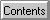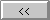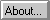# Observer Antenna Pattern

Pattern Loss
Most radio amateurs use antennas of three classes:
Steerable antennas
Near-isotropic antennas
Simple omnidirectional antennas such as a quarter-wave vertical or halfwave dipole or turnstile.
WinOrbit allows selection of antennas from these classes for calculation of pattern loss at the observer. For the first two types, the loss is constant, and set to 0 dB. Of course, a high-gain steerable antenna will have a gain (not loss) of 10-20dB, but this is constant, independent of the spacecraft position, provided the antenna is correctly pointed.
For the simple antennas, two choices are available: "turnstile" or "groundplane". Both types are assumed omnidirectional in the horizontal (azimuth) plane. The elevation plane loss is calculated from :
Loss(dB) = -.08 + 20 * Log(Sin([[pi]]/2 - Elevation) + .01)
for the groundplane (vertical type), and
Loss(dB) = -.08 + 20 * Log(Cos([[pi]]/2 - Elevation) + .01)
for the turnstile (horizontal) type. The .01 and .08 are used to prevent numerical overflow for angles near 0 or 90. As with the satellite antenna, the loss is limited to -40 dB under the worst case, since real antennas are rarely this directional.

Converted by Winhelp to Web